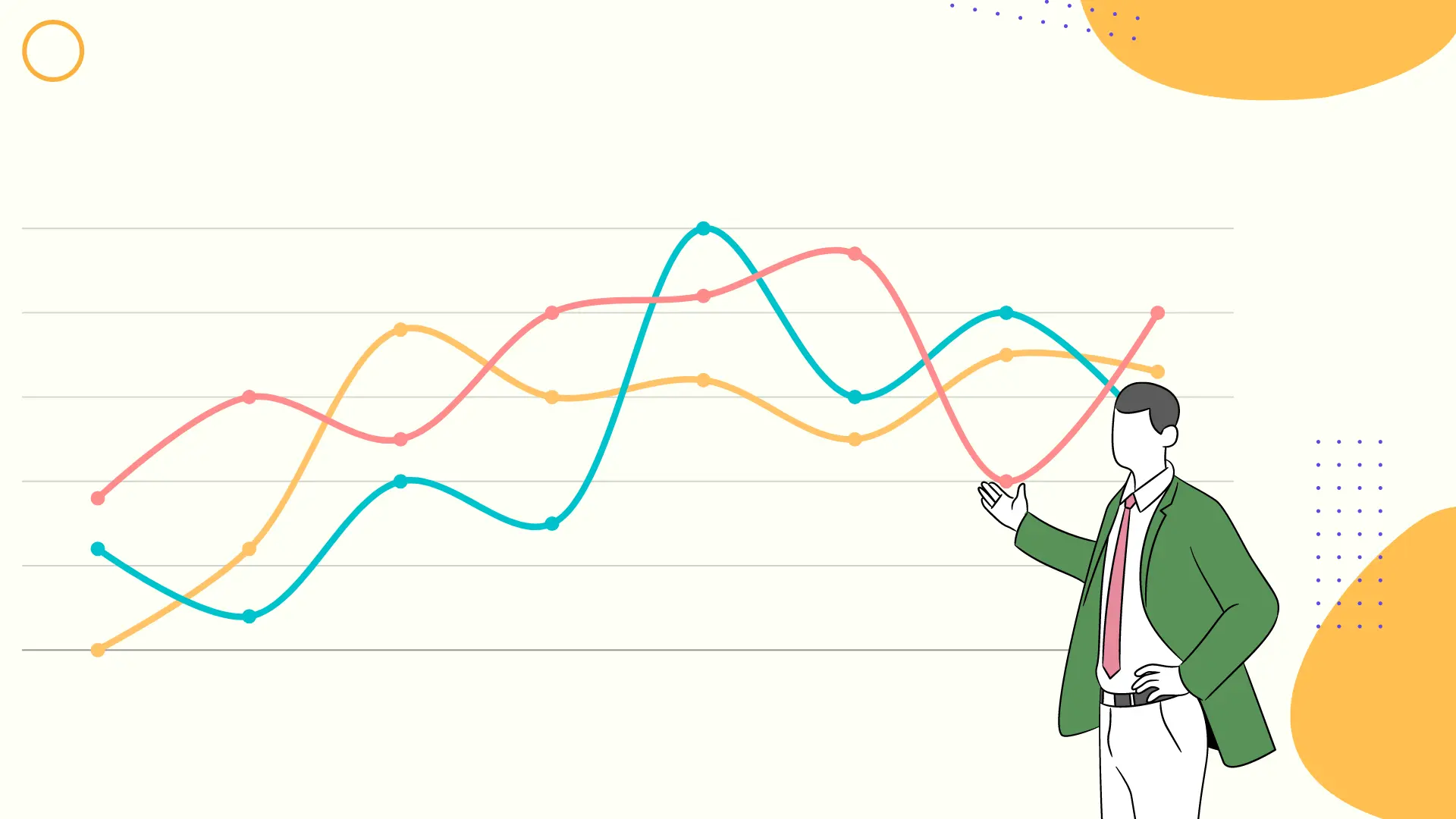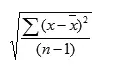# What Is Standard Deviation? The formula you need to know!

If you’ve ever taken a statistics course, the term “standard deviation” could frighten you. Thanks to Excel, calculating this statistic is much simpler than writing it down on paper since it can disclose details about datasets that averages cannot.

Financial professionals regularly utilize this since it can be used to assess risk in stock portfolios and is frequently used to calculate return on investment (ROI).

It may assist marketers in identifying dataset risks and variations that will eventually inform advertising selections.

## What exactly is the standard deviation?

Simply expressed, the mathematical concept of “standard deviation” refers to the measurement of variance in a collection of numbers. It may be used in marketing to account for significantly fluctuating expenses or sales. A data set’s standard deviation increases if the numbers are dispersed widely.

When choosing how much money should be spent on certain campaigns based on the standard deviation of the ROI, to provide one example, this statistic may assist in estimating risk.

## Excel Standard Deviation Calculation

Depending on whether you need to determine sample standard deviation or population standard deviation, one of Excel’s six standard deviation formulae will be used. Excel makes it simple to see this since each of the three calculations for population standard deviation includes a P (.P, PA, or P at the end of STDEV).

### 1. STDEV.S

This is the formula you will use to compute standard deviation in Excel if you are working with sample standard deviation and do not need to take text or logical values into consideration.

### 2. STDEVA

Alternatively, if text and logical values must be taken into consideration, utilize STDEVA, which will read TRUE logical values as 1, while text and FALSE logical values will be read as 0.

### 3. STDEV

Simply said, STDEV is the sample standard deviation calculation that is compatible with earlier versions of Excel (2007 and before). It is equivalent to STDEV. S.

### 4. STDEV, STDEVPA, and STDEV

You’ll mostly be using STDEV.S, STDEVA, or STDEV. You must consider all datasets for the whole population when calculating this.

In actuality, this may include far more data and be much less helpful than looking at a sample of the data, in which case you would use one of the formulae for the sample standard deviation.

You could still obtain sample standard deviations and apply them to the larger dataset if you needed to know the population standard deviation for whatever reason. These formulae  are unlikely to be necessary.

[STDEVP] has been replaced with one or more new functions that may provide enhanced accuracy and with names that better represent their purpose. Although this function is still accessible for backward compatibility, you should think about switching to the new functions going forward since this function could be removed from Excel in the future.

## Excel Standard Deviation Example

The most typical formulae to utilize are STDEV.S, STDEVA, or STDEV.

These algorithms compute standard deviation, particularly for a sample of a dataset, and the output will show how much the sample deviates from the mean (average) of the data.

The next step is to learn where to look for and how to utilize the standard deviation calculations in an Excel spreadsheet.

## Excel Standard Deviation Formulas

There are six standard deviations Excel formulae, as was described above, but you’ll only need one or two of them (depending on the version of Excel you use).

The Excel standard deviation formula for STDEV.S has the following syntax:

STDEV.S(number1,[number2],…)

The first number argument “corresponding to a sample of a population” is number1, which is essential. You may alternatively use a single array or a reference to an array instead of parameters separated by commas.

Number 2 is completely optional. You may use an array or a reference to an array in place of number arguments, or you can use up to 254 number parameters that relate to population sample sets or data.

Excel uses the following calculation for STDEV.S:The sample size is n, and x is the sample mean AVERAGE(number1,number2,…) Thankfully, applying this technique to data to compute this is a simple task made easier by Excel.

## Excel's standard deviation function

What steps must one take in order to locate the required Excel standard deviation function? Open Excel and go to the header for “Formulas” in the top navigation bar. A list of common formulae is listed under “More Functions” at the bottom of the second navigation bar for Excel versions released after 2007. Before scrolling down to the STDEV.S or any standard deviation formula you want, choose “More Functions,” then “Statistical.” Then, you may enter Number 1, Number 2, etc.

Even though Excel versions earlier than this one do not feature the STDEV.S formula, users may still find STDEV.

## Why Standard Deviation Matters to Marketers

Perhaps some marketing experts find this straightforward, or perhaps this is just a bunch of statistical jargon that seems complicated. In any case, it’s critical for marketers to learn this formula and these Excel features.

Averages for datasets are often discussed in marketing, and this may be instructive. However, it may also omit certain significant details that might have an effect on a campaign.

A dataset’s standard deviation might reveal hazards, volatility, or variability. It may reflect a campaign’s potential risk and return while an average might indicate a viable campaign to invest money in. Together, averages and standard deviations may provide a thorough analysis of a dataset, enabling marketers to make the best choices based on all available facts.

Consider two businesses with varying order averages as an illustration.

The typical order for one business is \$1,000. The average order value at Company Two is \$1,500.

However, business two’s deviation is \$500, whereas company one’s is just \$50. On the surface, Company Two seems to have a higher order average, but a closer look reveals that it is riskier.

## Conclusion

When presenting advertisers’ promising efforts and predicting their outcomes, averages might be useful. Averages, however, fail to reveal the risk and variability present in the data.

A greater average sales figure does not always indicate a wide range of unpredictability and danger. Marketers may gain from employing this statistical analysis in their own work, as it has long been used in finance to evaluate the risk of stock portfolios and aid experts in deciding where to invest or what ROI to anticipate.

By considering both averages and standard deviation while making judgments, they may make some unexpectedly valuable discoveries and stay away from dangerous, unpredictable campaigns or businesses.#### More to explore

AppSumo Sale! Get Fynzo Survey LIFETIME plan just for \$69 before the offer ends.

X## See survey maker in action!

Sign-Up and get a free trial of 14 days.

✅No credit card required ✅All features included ✅Book a demo